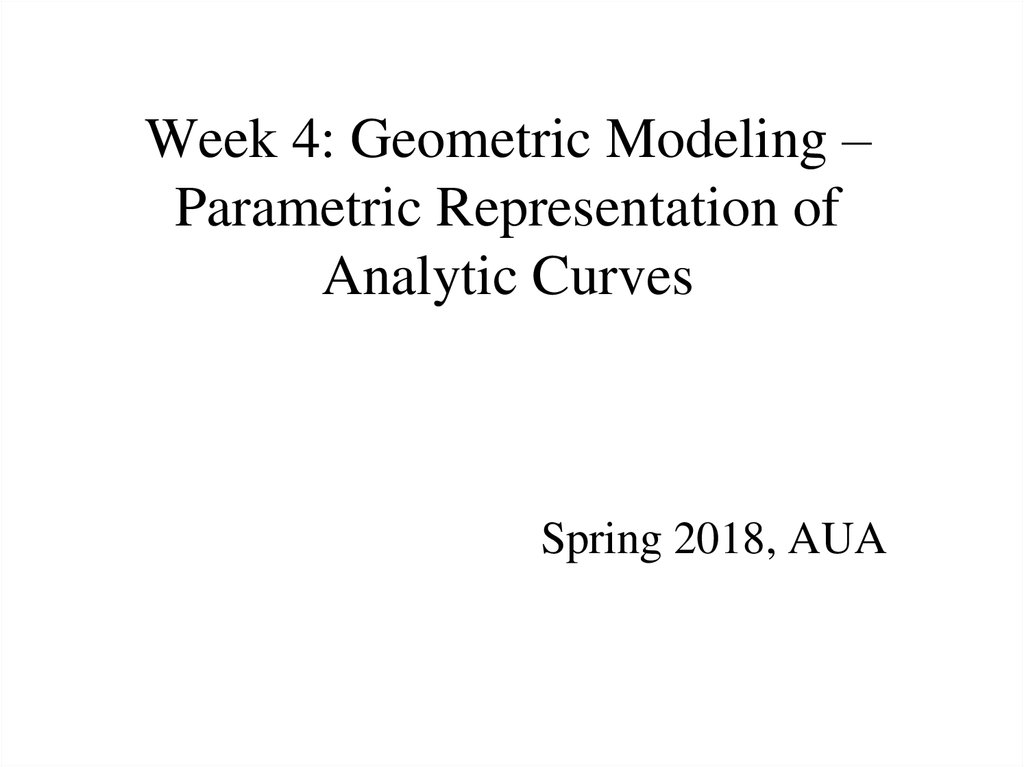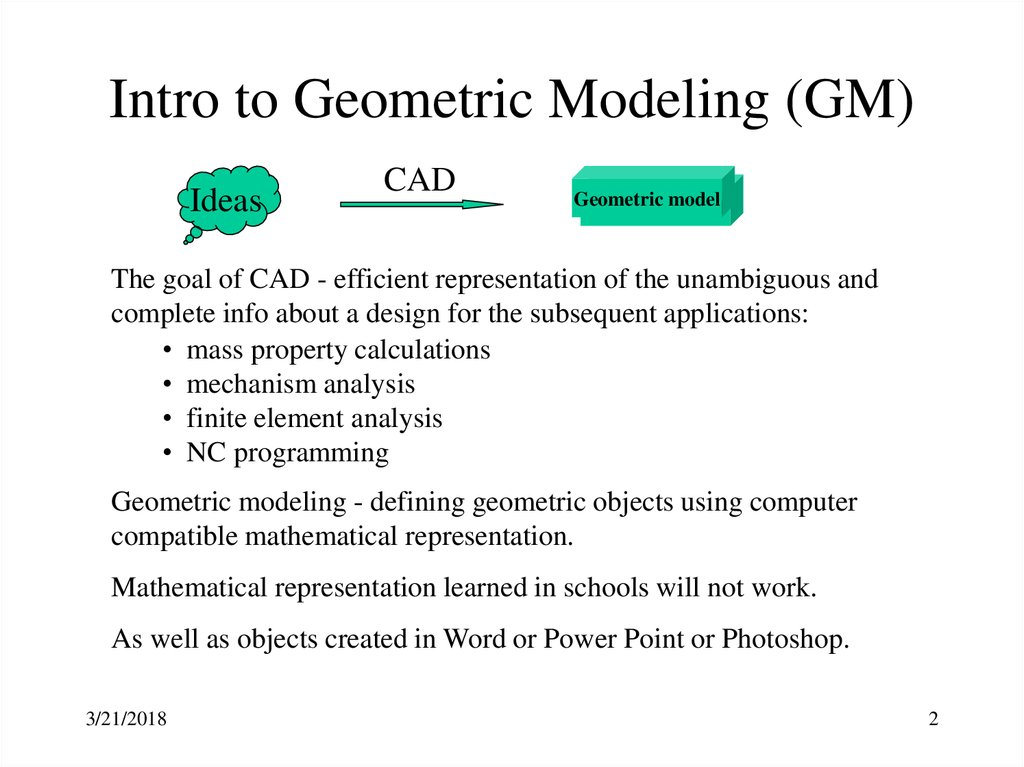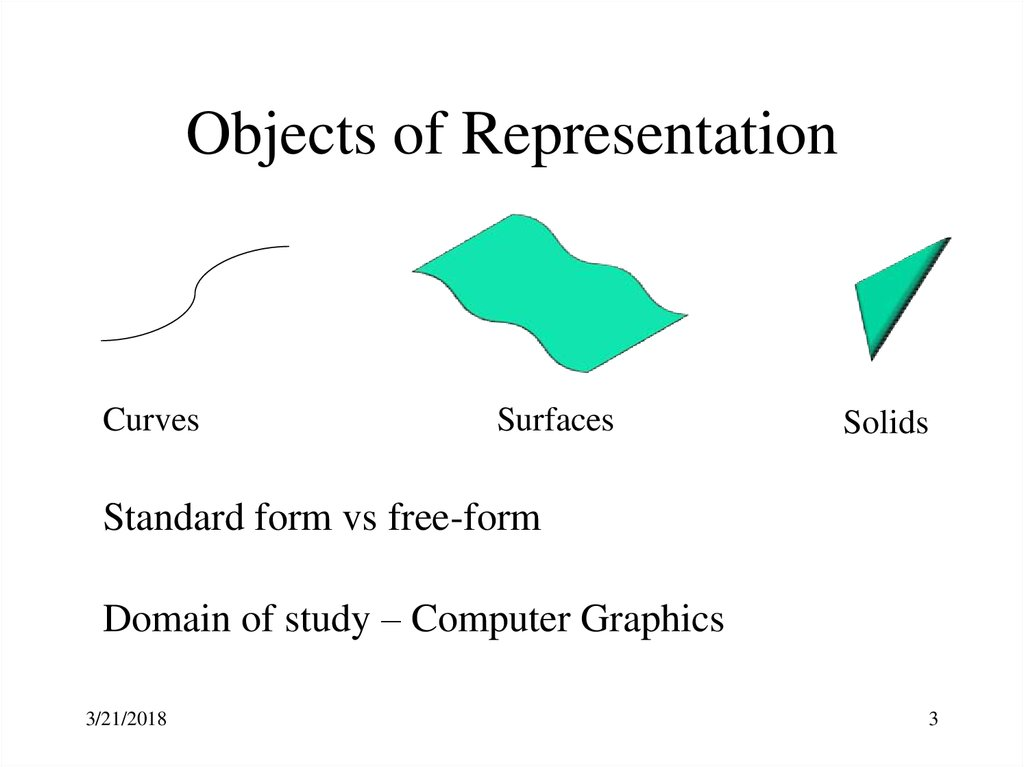# Intro to Geometric Modeling (GM)

## 1.

Week 4: Geometric Modeling –
Parametric Representation of
Analytic Curves
Spring 2018, AUA

## 2. Intro to Geometric Modeling (GM)

Ideas
CAD
Geometric
Geometricmodel
model
The goal of CAD - efficient representation of the unambiguous and
complete info about a design for the subsequent applications:
• mass property calculations
• mechanism analysis
• finite element analysis
• NC programming
Geometric modeling - defining geometric objects using computer
compatible mathematical representation.
Mathematical representation learned in schools will not work.
As well as objects created in Word or Power Point or Photoshop.
3/21/2018
2

## 3.

Objects of Representation
Curves
Surfaces
Solids
Standard form vs free-form
Domain of study – Computer Graphics
3/21/2018
3

## 4.

Types of Representation
Explicit
Representation
z=ax+by+cz+d
Implicit
Representation
ex+fy+gz+h=0
Parametric
Representation
x=a+bu+cw
y=d+eu+fw
z=g+hu+iw
The question is which one is computer compatible?
3/21/2018
4

## 5. Advantages of PR

• Get rid of dependency of the coordinates (X, Y, Z) from each
other.
3/21/2018
5

## 6. Advantages of PR

• Get rid of dependency of the coordinates (X, Y, Z) from each other.
• Can be extended to higher objects. (4th parameter )
3/21/2018
6

## 7. Advantages of PR

• Get rid of dependency of the coordinates (X, Y, Z) from each other.
• Can be extended to higher objects. (4th parameter )
• More DOF for controlling curves and surfaces.
3/21/2018
7

## 8. Advantages of PR

Get rid of dependency of the coordinates (X, Y, Z) from each other.
Can be extended to higher objects. (4th parameter )
More DOF for controlling curves and surfaces.
Transformations (distinct separation between shape and trans.
info).
R=7 circle at 0,0
3/21/2018
R=7 circle at 4,3
8

## 9. Advantages of PR

Get rid of dependency of the coordinates (X, Y, Z) from each other.
Can be extended to higher objects. (4th parameter )
More DOF for controlling curves and surfaces.
Transformations (distinct separation between shape and trans. info).
Vector – matrix multiplication (not good for you, good for comp).
3/21/2018
9

## 10. Advantages of PR

Get rid of dependency of the coordinates (X, Y, Z) from each other.
Can be extended to higher objects. (4th parameter )
More DOF for controlling curves and surfaces.
Transformations (distinct separation between shape and trans. info).
Vector – matrix multiplication (not good for you, good for comp.).
Bounded objects are represented with one-to one relationship.
3/21/2018
10

## 11. Advantages of PR

Get rid of dependency of the coordinates (X, Y, Z) from each other.
Can be extended to higher objects. (4th parameter )
More DOF for controlling curves and surfaces.
Transformations (distinct separation between shape and trans. info).
Vector – matrix multiplication (not good for you, good for comp.).
Bounded objects are represented with one-to one relationship.
No problems for slope calculation.
3/21/2018
11

## 12. Advantages of PR

Get rid of dependency of the coordinates (X, Y, Z) from each other.
Can be extended to higher objects. (4th parameter )
More DOF for controlling curves and surfaces.
Transformations (distinct separation between shape and trans. info).
Vector – matrix multiplication (not good for you, good for comp.).
Bounded objects are represented with one-to one relationship.
No problems for slope calculation.
Discretizing entities
3/21/2018
12

## 13.

Parametric Representation (PR)
X = f(t)
Y = g(t)
Z = h(t)
3/21/2018
13

Tangent vector
or
3/21/2018
14

## 15. PR of Analytic Curves

Analytic curves are defined by analytic equations
•Compact form for representation
•Simple computation of properties
3/21/2018
•Little practical use
•No local control
15

3/21/2018
16

n
0 ≤ L ≤ Lmax
3/21/2018
17

## 18. Parametric equation from NP Implicit Equation: Example

F(x, y) = 0
y
For
x
x2 + y2 - R2 = 0
R
x R cos 2 u, where 0 u 1
y R sin 2 u, where 0 u 1
Parametric equation :
P(u ) [ R cos 2 u, R sin 2 u ]T ,
3/21/2018
0 u 1
18

3/21/2018
19

+B/A
3/21/2018
20

## 21. Examples

• Find the equation and endpoints
of a line that passes through point
P1, parallel to an existing line,
and is trimmed by point P2.
• Relate the following CAD
commands to their mathematical
foundations:
– The command to measure the
angle between two
intersecting lines.
– The command to find the
distance between a point and
a line.
3/21/2018
P4
y
P5
n1
n1
P3
P2
P1
x
z
P2
P1
P4
P3
P2
P3
D
P4
n1
21